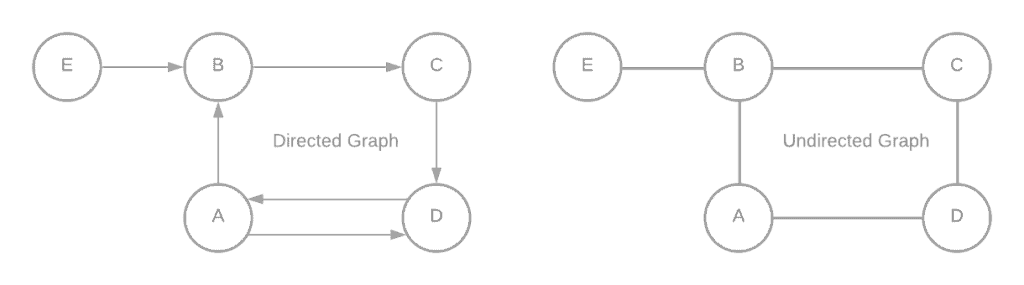## 1. Introduction

In this tutorial, we’re going to work with undirected graphs in order to extract their minimum spanning trees (MST) through Prim’s Algorithm. This is an essential algorithm in Computer Science and graph theory. Popular algorithms in graph theory include Djikstra’s shortest path algorithm, Kruskal’s algorithm, and many search algorithms.

## 2. Concepts

There are numerous phenomena in Computer Science that are best represented through graphs. A graph can be seen as a model of different nodes (also called vertices or points) connected through edges (also called links or lines).

A subset of these that do not specify a direction between nodes is called “undirected graphs”. Furthermore, one of the most common occurrences of these is computer networks.

However, we should think more broadly of these omnipresent mathematical structures. We should note their importance in the fields of linguistics and natural language processing, logistics, geometry, neurology, sociology, chemistry, and many others. Think of how we represent molecules, geometric shapes,  relations between words, or shipping routes:Consequently, there exists a wide array of common problems in graphs that have been thoroughly examined by mathematicians and computer scientists.

For example, one of these problems is finding the shortest path to reach all nodes in a graph. This path is called the “minimum spanning tree”.  Accordingly, various algorithms have the purpose of finding this path, and one of the most commonly used is Prim’s.  This is a greedy method of determining the minimum spanning tree across an undirected graph. We can note that the word “greedy” designates an algorithm that will make the best choice at every step to find the optimal solution to the problem.

## 3. Implementation

This algorithm can be implemented in three main steps, which we’ll then decompose.

1. Pick any vertex in our graph. This will be the starting point in our tree.
2. Iterate through all of the edges that are not yet in our tree but that are connecting to the nodes of our tree and pick the smallest one. We’ll add this minimum-weight edge to our tree.
3. Check if our tree contains all of the nodes. If not, repeat step 2.

We say that this algorithm is greedy because we pick the best node.

Furthermore, we can further decompose this into multiple steps using pseudo-code.This procedure can be visualized in the following animation:## 4. Complexity

The time complexity analysis of this algorithm for V vertices and E edges in a given graph is based upon the data structures with you wish to implement it:

• For a binary heap and a list, the complexity is• In the case of a Fibonacci heap and a list,  we get a more complex equation :• An adjacency matrix would give us a complexity of## 5. Conclusion

In this article, we discussed Prim’s algorithm for finding the Minimum Spanning Tree in graphs.